Search by Topic

Resources tagged with Mathematical modelling similar to Universal Time, Mass, Length:

Filter by: Content type:
Age range:
Challenge level:

There are 72 results

Broad Topics > Using, Applying and Reasoning about Mathematics > Mathematical modellingPredator - Prey Systems

Age 16 to 18 Challenge Level:

See how differential equations might be used to make a realistic model of a system containing predators and their prey.The Not-so-simple Pendulum 1

Age 16 to 18 Challenge Level:

See how the motion of the simple pendulum is not-so-simple after all.Ramping it Up

Age 16 to 18 Challenge Level:

Look at the calculus behind the simple act of a car going over a step.Population Dynamics - Part 5

Age 16 to 18 Challenge Level:

Fifth in our series of problems on population dynamics for advanced students.Population Dynamics - Part 1

Age 16 to 18 Challenge Level:

First in our series of problems on population dynamics for advanced students.Population Dynamics - Part 4

Age 16 to 18 Challenge Level:

Fourth in our series of problems on population dynamics for advanced students.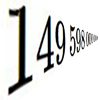Big and Small Numbers in Physics - Group Task

Age 16 to 18 Challenge Level:

Work in groups to try to create the best approximations to these physical quantities.Physnrich

Age 14 to 18 Challenge Level:

PhysNRICH is the area of the StemNRICH site devoted to the mathematics underlying the study of physicsPopulation Ecology Using Probability

Age 16 to 18 Challenge Level:

An advanced mathematical exploration supporting our series of articles on population dynamics for advanced students.Population Dynamics - Part 6

Age 16 to 18 Challenge Level:

Sixth in our series of problems on population dynamics for advanced students.Population Dynamics - Part 2

Age 16 to 18 Challenge Level:

Second in our series of problems on population dynamics for advanced students.Population Dynamics Collection

Age 16 to 18 Challenge Level:

This is our collection of tasks on the mathematical theme of 'Population Dynamics' for advanced students and those interested in mathematical modelling.Guessing the Graph

Age 14 to 16 Challenge Level:

Can you suggest a curve to fit some experimental data? Can you work out where the data might have come from?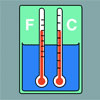Stemnrich - the Physical World

Age 11 to 16 Challenge Level:

PhysNRICH is the area of the StemNRICH site devoted to the mathematics underlying the study of physicsBranching Processes and Extinction

Age 16 to 18 Challenge Level:

An advanced mathematical exploration supporting our series of articles on population dynamics for advanced students.Modelling Assumptions in Mechanics

Age 16 to 18

An article demonstrating mathematically how various physical modelling assumptions affect the solution to the seemingly simple problem of the projectile.Population Dynamics - Part 3

Age 16 to 18 Challenge Level:

Third in our series of problems on population dynamics for advanced students.Chemnrich

Age 14 to 18 Challenge Level:

chemNRICH is the area of the stemNRICH site devoted to the mathematics underlying the study of chemistry, designed to help develop the mathematics required to get the most from your study. . . .Engnrich

Age 16 to 18 Challenge Level:

engNRICH is the area of the stemNRICH Advanced site devoted to the mathematics underlying the study of engineeringBionrich

Age 14 to 18 Challenge Level:

bioNRICH is the area of the stemNRICH site devoted to the mathematics underlying the study of the biological sciences, designed to help develop the mathematics required to get the most from your. . . .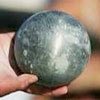Model Solutions

Age 16 to 18 Challenge Level:

How do these modelling assumption affect the solutions?The Wrong Stats

Age 16 to 18 Challenge Level:

Why MUST these statistical statements probably be at least a little bit wrong?Pdf Stories

Age 16 to 18 Challenge Level:

Invent scenarios which would give rise to these probability density functions.Population Dynamics

Age 16 to 18 Challenge Level:

This problem opens a major sequence of activities on the mathematics of population dynamics for advanced students.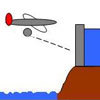Dam Busters 1

Age 16 to 18 Challenge Level:

At what positions and speeds can the bomb be dropped to destroy the dam?Designing Table Mats

Age 11 to 16 Challenge Level:

Formulate and investigate a simple mathematical model for the design of a table mat.Over-booking

Age 16 to 18 Challenge Level:

The probability that a passenger books a flight and does not turn up is 0.05. For an aeroplane with 400 seats how many tickets can be sold so that only 1% of flights are over-booked?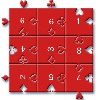Impuzzable

Age 16 to 18

This is about a fiendishly difficult jigsaw and how to solve it using a computer program.Age 16 to 18 Challenge Level:

This is the section of stemNRICH devoted to the advanced applied mathematics underlying the study of the sciences at higher levels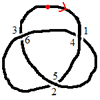Drawing Doodles and Naming Knots

Age 7 to 18

This article for students introduces the idea of naming knots using numbers. You'll need some paper and something to write with handy!What's a Knot?

Age 7 to 16 Challenge Level:

A brief video explaining the idea of a mathematical knot.Escalator

Age 14 to 16 Challenge Level:

At Holborn underground station there is a very long escalator. Two people are in a hurry and so climb the escalator as it is moving upwards, thus adding their speed to that of the moving steps. . . .
Concrete Calculation

Age 14 to 16 Challenge Level:

The builders have dug a hole in the ground to be filled with concrete for the foundations of our garage. How many cubic metres of ready-mix concrete should the builders order to fill this hole to. . . .The Legacy

Age 14 to 16 Challenge Level:

Your school has been left a million pounds in the will of an ex- pupil. What model of investment and spending would you use in order to ensure the best return on the money?Spot the Card

Age 14 to 16 Challenge Level:

It is possible to identify a particular card out of a pack of 15 with the use of some mathematical reasoning. What is this reasoning and can it be applied to other numbers of cards?Where to Land

Age 14 to 16 Challenge Level:

Chris is enjoying a swim but needs to get back for lunch. If she can swim at 3 m/s and run at 7m/sec, how far along the bank should she land in order to get back as quickly as possible?Shaping the Universe III - to Infinity and Beyond

Age 11 to 16

The third installment in our series on the shape of astronomical systems, this article explores galaxies and the universe beyond our solar system.Chocolate 2010

Age 14 to 16 Challenge Level:

First of all, pick the number of times a week that you would like to eat chocolate. Multiply this number by 2...Rocking Chairs, Railway Games and Rayboxes

Age 5 to 18

In this article for teachers, Alan Parr looks at ways that mathematics teaching and learning can start from the useful and interesting things can we do with the subject, including. . . .FA Cup

Age 16 to 18 Challenge Level:

In four years 2001 to 2004 Arsenal have been drawn against Chelsea in the FA cup and have beaten Chelsea every time. What was the probability of this? Lots of fractions in the calculations!Maximum Flow

Age 16 to 18 Challenge Level:

Given the graph of a supply network and the maximum capacity for flow in each section find the maximum flow across the network.The Mean Game

Age 16 to 18

Edward Wallace based his A Level Statistics Project on The Mean Game. Each picks 2 numbers. The winner is the player who picks a number closest to the mean of all the numbers picked.Twenty20

Age 7 to 16 Challenge Level:

Fancy a game of cricket? Here is a mathematical version you can play indoors without breaking any windows.In Constantly Passing

Age 14 to 16 Challenge Level:

A car is travelling along a dual carriageway at constant speed. Every 3 minutes a bus passes going in the opposite direction, while every 6 minutes a bus passes the car travelling in the same. . . .Investigating Epidemics

Age 11 to 16 Challenge Level:

Simple models which help us to investigate how epidemics grow and die out.Bird-brained

Age 16 to 18 Challenge Level:

How many eggs should a bird lay to maximise the number of chicks that will hatch? An introduction to optimisation.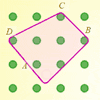Elastic Maths

Age 14 to 18

How do you write a computer program that creates the illusion of stretching elastic bands between pegs of a Geoboard? The answer contains some surprising mathematics.An Introduction to Computer Programming and Mathematics

Age 16 to 18

This article explains the concepts involved in scientific mathematical computing. It will be very useful and interesting to anyone interested in computer programming or mathematics.Stringing it Out

Age 14 to 16 Challenge Level:

Explore the transformations and comment on what you find.Cushion Ball

Age 16 to 18 Challenge Level:

The shortest path between any two points on a snooker table is the straight line between them but what if the ball must bounce off one wall, or 2 walls, or 3 walls?